## Example Questions

← Previous 1

### Example Question #1 : How To Find The Volume Of A Cone

An empty tank in the shape of a right solid circular cone has a radius of r feet and a height of h feet. The tank is filled with water at a rate of w cubic feet per second. Which of the following expressions, in terms of r, h, and w, represents the number of minutes until the tank is completely filled?

π(r2)(h)/(180w)

π(r2)(h)/(20w)

20w/(π(r2)(h))

π(r2)(h)/(60w)

180w/(π(r2)(h))

π(r2)(h)/(180w)

Explanation:

The volume of a cone is given by the formula V = (πr2)/3. In order to determine how many seconds it will take for the tank to fill, we must divide the volume by the rate of flow of the water.

time in seconds = (πr2)/(3w)

In order to convert from seconds to minutes, we must divide the number of seconds by sixty. Dividing by sixty is the same is multiplying by 1/60.

(πr2)/(3w) * (1/60) = π(r2)(h)/(180w)

### Example Question #2 : How To Find The Volume Of A Cone

A cone has a base radius of 13 in and a height of 6 in.  What is its volume?

1352π in3

338π in3

1014π in3

4394π in3

338π in3

Explanation:

The basic form for the volume of a cone is:

V = (1/3)πr2h

For this simple problem, we merely need to plug in our values:

V = (1/3)π13* 6 = 169 * 2π = 338π in3

### Example Question #3 : How To Find The Volume Of A Cone

A cone has a base circumference of 77π in and a height of 2 ft.  What is its approximate volume?

2964.5π in3

71,148π in3

8893.5π in3

142,296π in3

11,858π in3

11,858π in3

Explanation:

There are two things to be careful with here.  First, we must solve for the radius of the base. Secondly, note that the height is given in feet, not inches. Notice that all the answers are in cubic inches. Therefore, it will be easiest to convert all of our units to inches.

First, solve for the radius, recalling that C = 2πr, or, for our values 77π = 2πr. Solving for r, we get r = 77/2 or r = 38.5.

The height, in inches, is 24.

The basic form for the volume of a cone is: V = (1 / 3)πr2h

For our values this would be:

V = (1/3)π * 38.52 * 24 = 8 * 1482.25π = 11,858π in3

### Example Question #4 : How To Find The Volume Of A Cone

What is the volume of a right cone with a diameter of 6 cm and a height of 5 cm?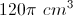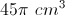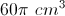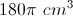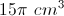Explanation:

The general formula is given by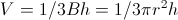, where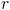= radius and= height.

The diameter is 6 cm, so the radius is 3 cm.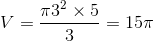### Example Question #1 : Cones

There is a large cone with a radius of 4 meters and height of 18 meters. You can fill the cone with water at a rate of 3 cubic meters every 25 seconds. How long will it take you to fill the cone?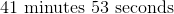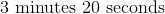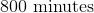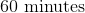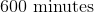Explanation:

First we will calculate the volume of the cone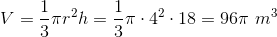Next we will determine the time it will take to fill that volume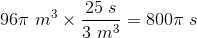We will then convert that into minutes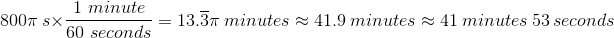### Example Question #6 : How To Find The Volume Of A Cone

Find the volume of a cone with a radius ofand a height of.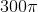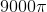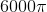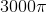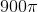Explanation:

Write the formula to find the volume of a cone.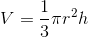Substitute the known values and simplify.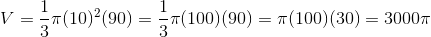### Example Question #7 : How To Find The Volume Of A Cone

Find the volume of a cone with radius 3 and height 5.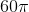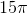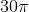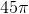Explanation:

To solve, simply use the formula for the volume of a cone. Thus,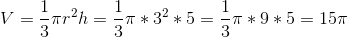To remember the formula for volume of a cone, it helps to break it up into it's base and height. The base is a circle and the height is just h. Now, just multiplying those two together would give you the formula of a cylinder (see problem 3 in this set). So, our formula is going to have to be just a portion of that. Similarly to volume of a pyramid, that fraction is one third.

### Example Question #8 : How To Find The Volume Of A Cone

Find the area of a cone whose radius is 4 and height is 3.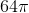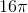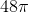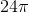Explanation:

To solve, simply use the formula for the area of a cone. Thus,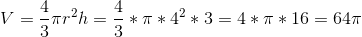### Example Question #9 : How To Find The Volume Of A Cone

The volume of a right circular cone is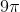. If the cone's height is equal to its radius, what is the radius of the cone?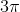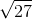Explanation:

The volume of a right circular cone with radiusand heightis given by: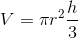Since the height of this cone is equal to its radius, we can say: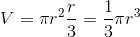Now, we can substitute our given volume into the equation and solve for our radius.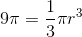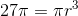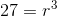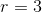### Example Question #10 : How To Find The Volume Of A Cone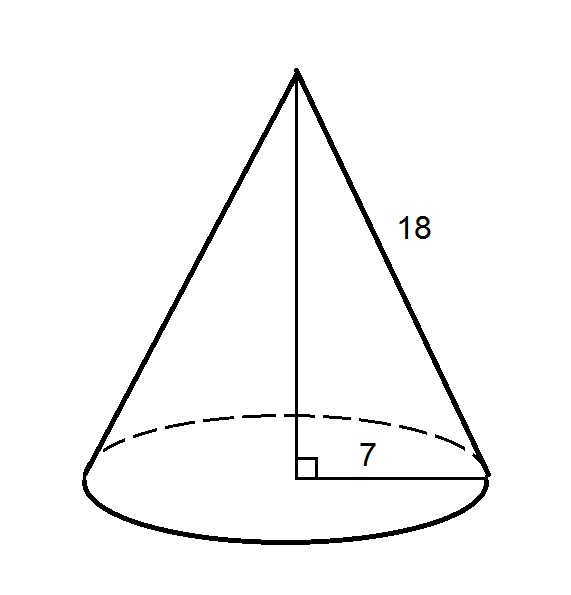The above is a right circular cone. Give its volume.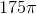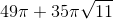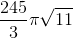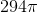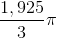Explanation:

The volume of a right circular conecan be calculated from its heightand the radiusof its base using the formula.

We are given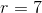, but not.,, and the slant heightof a right circular cone are related by the Pythagorean Theorem: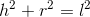Setting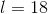and, substitute and solve for: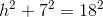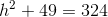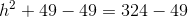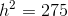Taking the square root of both sides and simplifying the radical: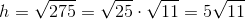Now, substitute forandand evaluate: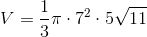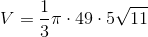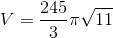← Previous 1